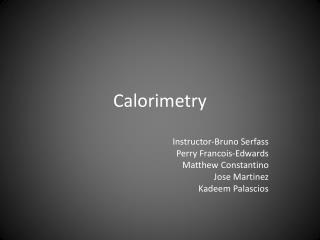DownloadDownload PresentationCalorimetry

Calorimetry

Download PresentationCalorimetry

- - - - - - - - - - - - - - - - - - - - - - - - - - - E N D - - - - - - - - - - - - - - - - - - - - - - - - - - -
Presentation Transcript

1. Calorimetry Instructor-Bruno Serfass Perry Francois-Edwards Matthew Constantino Jose Martinez KadeemPalascios

2. Heat Conductivity • Heat conductivity is how fast a material can transfer heat from one end to another • This experiment measures heat conductivity and how fast different metals transfer heat • We transferred heat from boiling water (100 degrees C) through a tube that runs underneath the poles • The orange and yellow poles are different metals • We tested the temperature of the poles with thermometers in our hands

3. Heat Conductivity Results • Red is copper • Blue is aluminum • Green is brass • Yellow is lead • Copper transferred heat from the water the fastest • Copper is a good conductor of heat (energy) • So if you want to conduct heat fast you should use copper

4. Heat Capacity • We tested different masses and specific heats to see which ball had the most heat capacity • We multiplied the specific heat which is the amount of heat that must be absorbed or lost for that substance to change it’s temperature by 1 degree by the mass in grams in the balls • Mass: Specific Heat Heat Capacity • Zinc: 24 g .092 2.208 • Glass: 10 g .16 1.6 • Lead: 45 g .031 1.395 • Iron: 28 g .105 2.94 • Brass:40g .092 2.76 • Heat Capacity= Mass x Specific Heat

5. Heat Capacity Results • Two of the metal balls melted through the the wax tablet. • So the two balls that went through the tablet had the greatest heat capacity. Those two balls were the Iron and Brass. • It also depended on the mass of the ball because if it has a higher mass and a higher specific heat, it’s heat capacity will be increased as well.

6. Calorimeter Experiment (small scale) • The objective of this experiment was to find the energy that was transferred from a piece of brass into the water in the calorimeter by recording the change in temperature. • We used this formula: q=mc(T2-T1) • q=energy or heat • m=mass • c=specific heat • T2-T1= change in temperature

7. Calorimeter Experiment (small scale) • The piece of brass • The calorimeter • Thermometers • In the experiment we put some heated brass in a small calorimeter to see the basics of how a calorimeter works

8. Calorimeter Experiment

9. Dark Matter Calorimeter • With the calorimeter here at UC Berkeley we used it to record “events”, when a particle interactsinsidethe germanium crystal. • During the experiment we needed to refill the liquid helium bath, which Perry volunteered for, and had a bit of a technical difficulty

10. Calorimeter

11. Safety First

12. Measurements of Energy • Measurements of energy are joules (j), calories (cal), and electron volts (eV) • 1 eV = 1.6x10^-19 j • We convert electron volts into joules to make it compatible with the units for specific heat • Liquid helium is cold enough to be used to cool down germanium crystal which allows the temperature to be measured in more reasonable measurements

13. Dark Matter • A pulse is used to detect the particle based on the energy that it transfers into the germanium crystal (right) • An event is a pulse,there are 350,000 every 12 h • pulse is1-a few hundreds keV

14. Dark Matter(cont.) • The actual calorimeter that is currently being used in the search for dark matter particles is located 2000 ft. below sea level so the amount of events from known particles that it records is cut dramatically and hopefully raise the chances of detecting dark matter